• 十年踪迹
• 8578 访问

# JS1K 2015: Defender

2015年3月16号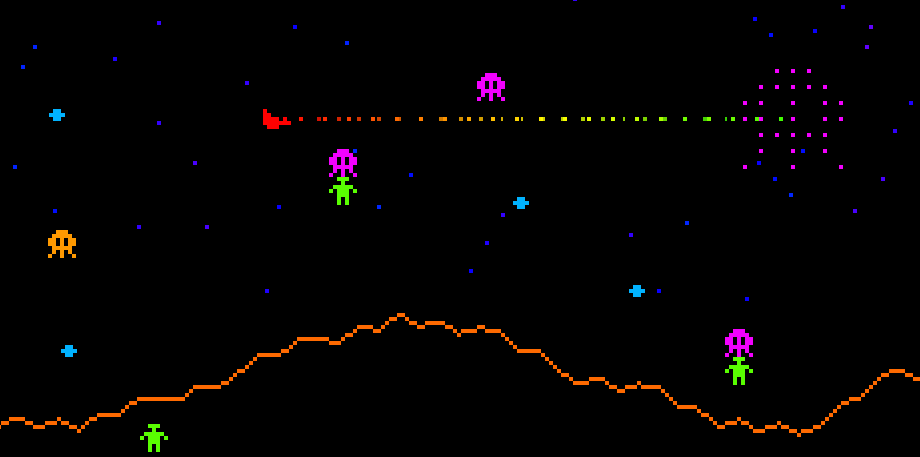• 人工智能：外星侵略者捕获并绑架人类，被绑架的人类突变返回陆地追逐玩家，外星侵略者和突变体可以向人类发射导弹。
• 可以无限滚动的场景和视差星域。
• 精灵，激光，爆炸和各种变形效果。

## 为打包器写代码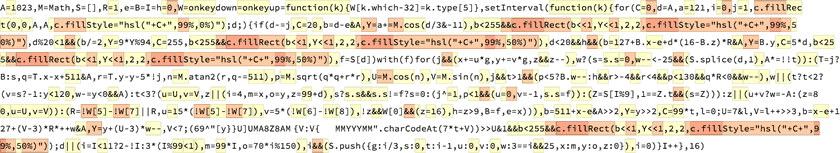Defender 源代码的打包模式

``````X<255&&c.fillRect(X<<1,Y<<1,2,2,c.fillStyle="hsl("+C+",99%,50%)")
``````

## 绘制精灵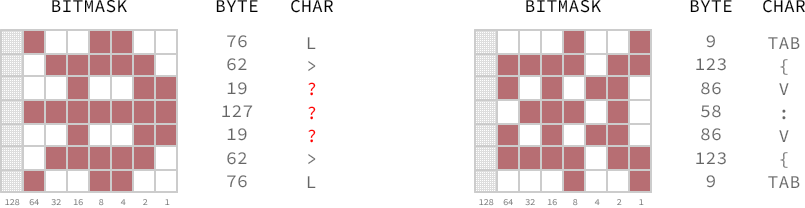``````var myEncodedSprites = '[y}}U]UMA8Z8AMEMYYMEE {V:V{  {V:V{ ';
``````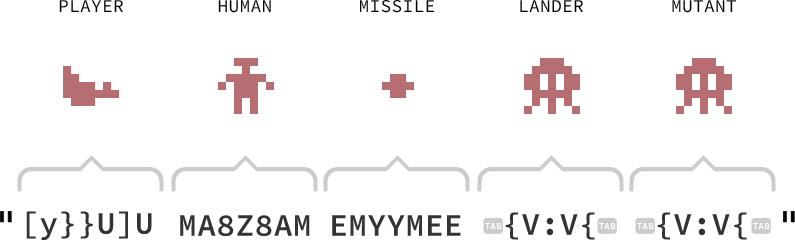demo中使用的精灵是用他们的行列数据转置器生成的，所以他们看起来像是旋转过了一样，我这样做来满足可变宽的精灵（原始游戏中有一个更宽的玩家太空船）来避免去处理8比特所带来的问题。但是不幸的是，我并没有预留足够的空间来这样做。

``````for(l=55;X=l&7,Y=l>>3,l--;)(69^'[y}}U]U'.charCodeAt(Y))>>X&1&&c.fillRect(Y,X,1,1)
``````

### 变形和爆炸效果

``````// t = 0      // Sprite                     (0 = player, 1 = human, 2 = missle)
// d = 1      // Direction                  (-1 = left, 1 = right)
// w = 1      // Warp / Explosion factor    (1 = normal)
// x = 100    // X position
// y = 100    // Y position

for(l=55;U=l&7,V=l>>3,X=x+d*(V-3)*w,Y=y+(U-3)*w,l--;)
(69^'[y}}U]UMA8Z8AMEMYYMEE'.charCodeAt(t*7+V))>>U&1&&c.fillRect(X,Y,1,1)
``````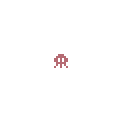## 绘制背景

Defender中的背景图包含了一个崎岖的波浪起伏的地面和一个视差滚动的星域，然而这些元素只是纯粹的装饰品，将他们中的每一个从我的作品中抽离出去都会使得这个游戏死气沉沉的，所以我需要将他们加入到这个游戏中去。

``````for(Y=127,X=1023;X;Y+=Math.cos(X--/5&-11))c.fillRect(X,Y|0,1,1)
``````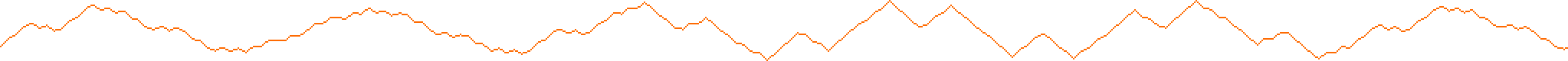## 最后是代码

``````for(_='s=KC=GL=B&&Q<4QP,X=`W[_=1=Math.cos(0%)")25)):c.fill,onkey99 s. {V:V{   -H+127+||(w--<1&A,Y),0,(q=-511+(E[d])Rect(x-x+511&Ar==function(k){E._k.which-32]=,Style="hsl("+C+", %,-y,psqrt(q*q+r*rnatan2(r,qunvsin(nX<5QX<,Y<,2,25H=\$=J=D,A023,E=[]down1}up0},setInterval(Wfor(F27,BGd=A,A;d--;){if(G`d-H=F+d/5&-11,G-F,X/=2,Y *F< Q,JQ(G5*d`(16-z)*d*Dx=y,d<Qwith(for(l=55,x+=u*g,y+=v*g,zQz--,w?(KK<-Q(A*=t>E.splice(d,1)yp<5?t>1Q:JQ-rPrPp30Qq*DQ,t?t<2?(v=s?-1:y4yQ(B5,t<3?zu+v?w=-A:(z= (zB3,O=x,N=y,z= +dsQ!wQ!(sQs!=?y-8pQ(x=x,y-=r,K):KtPE[\$%d],t=K0(H=x+u*2,I=!_5]-!_7],D=I||D,u-=I?u*I<Q-I/2:u/,y+=_8]?y40:yQ-!!_6],zz6*_0]J=z>9));U=l&7,V=l>>3`x(U-3)*D*++w=y+*(V-3G *t,l--;(69^"[y}}U]UMA8Z8AMEMYYMEE".charCodeAt(7*t+V))>>U&1Q);dB\$1?2-!\$:\$% Q4,N=70*L%24O= *\$LQE.push({g:L/4,t:L-1,w:L>3Q,s:Bu:v:z:x:O,y:N})}\$++},16)';g=/[- -_`PQBGK]/.exec(_);)with(_.split(g))_=join(shift());eval(_)
``````

…当然了这里有全部注释的源代码：

``````/*
* Defender: 1024 by Keith Clark
*
* A 1K game inspired by the classic arcade shooter Defender. Written
* for the 2015 JS1K competition.
*
* Save the humans from alien abduction! Pilot your ship and fire its
* laser at enemy landers before they abduct and transform humans into
* mutants programmed to hunt you down!
*
* Use the arrow keys to fly and space to fire.
*
* web: keithclark.co.uk | tweet: @keithclarkcouk
*/

// Initialise any globals

H =                                                 // Camera position
\$ =                                                 // Game frame ticks
J = 0,                                              // Player fire flag
D = 1,                                              // Player direction
A = 1023,                                           // World width
E = [],                                             // Entity stack

// Handle user input
// W = fire           [space bar]
// W = thrust left    [left arrow]
// W = thrust right   [right arrow]
// W = climb          [up arrow]
// W = dive           [down arrow]

onkeydown = function(k) {                           // key down state handler
W[k.which - 32] = 1;                                 // set key flag
},
onkeyup = function(k) {                             // key up state handler
W[k.which - 32] = 0;                                 // clear key flag
},

// Game loop

setInterval(W = function(k) {
for (
F = 127,                                           // mountain range Y start position
c.fillRect(L = 0, C = 0,d = A, A,                  // clear the screen
c.fillStyle = "hsl(" + C + ",99%,0%)"
)
;
d--                                                // for 1023 cycles...
;
) {
if (

// Draw a mountain pixel

C = 25,                                             // set colour to brown
X = d - H & A,                                      // set pixel X position
Y = F += Math.cos(d / 5 & -11),                     // set pixel Y position
X < 255 &&                                             // if the pixel is in the viewport
c.fillRect(X << 1, Y << 1, 2, 2,                        // plot the pixel
c.fillStyle = "hsl(" + C + ",99%,50%)"
),

// Draw a starfield pixel

C = -F,                                             // set colour to dark blue
X /= 2,                                             // half mountain X pixel (simple parallax)
Y = 199 * F & A,                                    // set pixel Y position
Y < 99 && X < 255 &&                                   // if the pixel is in the viewport
c.fillRect(X << 1, Y << 1, 2, 2,                        // plot the pixel
c.fillStyle = "hsl(" + C + ",99%,50%)"
),

// Draw a player lazer pixel

J && (
C = 5 * d,                                        // set colour spread from red to green
X = (16 - E.z) * d * D - H + 127 + E.x & A, // set X position
Y = E.y,                                       // set Y position matches player Y position
d < 25 && X < 255 &&                                 // if the pixel is in the viewport
c.fillRect(X << 1, Y << 1, 2, 2,                      // plot the pixel
c.fillStyle = "hsl(" + C + ",99%,50%)"
)
),

E[d]                                                // check we have an entity to process

// Game logic

) with (E[d]) for (
l = 55,                                             // entity sprite has is (8 * 7 - 1) bits
x += u * g,                                         // increment entity X position
y += v * g,                                         // increment entity Y position
z && z--,                                           // decrement entity timer (used for fire rate / bullet life etc.)

w ?                                                 // if this entity warping (w>0) or exploding (w<0)
(
s = s.s = 0,                                       // clear link with any other entity (lander -> human -> lander)
w-- < -25 && (                                     // decrement warp / explosion counter. If explosion has ended...
A *= t > 0,                                         // stop the game if the entity is the player
E.splice(d, 1)                                      // remove the dead entity from the game
)
)
:                                                   // the entity is out of warp and "in play"
(
q = -511 + (E.x - x + 511 & A),                 // get x delta to player (-511 to +511)
r = E.y - y,                                    // get y delta to player
p = Math.sqrt(q * q + r * r),                      // get distance to player
n = Math.atan2(r, q),                              // get angle to player
p < 5 ?                                            // if entity has hit player
t > 1 && E.w--                                   // if entity is not a human, explode player
:
J && -r < 4 && r < 4 &&                          // if player is firing and entity inline with player
p < 130 && q * D < 1 && w--,                        // if entity is in front of player, explode entity
t ?
t < 2 ?                                          // if entity is a HUMAN
(
v = s ?                                         // if grabbed by a lander
-1                                               // make human climb (the lander will actually chase it)
:                                               // if not grabbed by a lander
y < 140,                                         // if human is in free air, fall to earth
y < 1 && (L = 5, w--)                           // if human was captured, kill it and spawn... a... MUTANT!
)
:
t < 3 ?                                        // if entity is a BULLET
z || (                                         // if entity timer has reached 0
u + v ?                                         // and it's moving
w = -A                                        // instantly destroy it
:
(                                        // if it's not moving, it's a new bullet
u = Math.cos(n),                       // set X direction to track player
v = Math.sin(n),                       // set Y direction to track player
z = 99                                    // set bullet life
)
)
:
(                                           // if entity is a LANDER or a MUTANT
u = Math.cos(n),                             // set X direction to track player
v = Math.sin(n),                             // set X direction to track player
z || (                                          // if the timer has reached 0
L = 3,                                        // spawn a BULLET
O = x,                                        // spawn at entity X position
N = y,                                        // spawn at entity Y position
z = 99 + d                                    // reset the entity firing timer
),

s && !s.w && !(s.s && s.s != E[d]) ?         // if tracking a human (a lander)
(
q = -511 + (s.x - x + 511 & A),             // get x delta to human (-511 to +511)
r = s.y - 8 - y,                            // get y delta to human
p = Math.sqrt(q * q + r * r),               // get distance to human
n = Math.atan2(r, q),                       // get angle to human
u = Math.cos(n),                            // set X direction to track human
v = Math.sin(n),                            // set Y direction to track human
p < 1 && (                                  // if lander is grabbing human
x = s.x,                                     // align x values (prevents rounding issues)
y -= r,                                      // align y values (prevents rounding issues)
s.s = E[d]                                   // link the human to the lander
)
)
:
s = t < 4 && E[\$ % d],                       // if not tracking and not a mutant - pick an entity...
s.t == 1 || (s = 0)                             // if it's a human, track it
)
:
(                                             // if entity is the PLAYER
H = x + u * 2,                                 // set the camera
I = !W - !W,                             // determine X input
D = I || D,                                    // set player direction
u -= I ? u * I < 25 && -I / 2 : u / 25,        // set player X velocity
y += W ? y < 140 : y && -!!W,            // set player Y position
z || (z = 16 * W),                          // is player able to fire?
J = z > 9                                      // set player firing flag
)
)
;

// Entity rendering

U = l & 7,                                            // get sprite bit column
V = l >> 3,                                           // get sprite bit row
X = x - H + 127 + (V - 3) * D * ++w & A,              // pixel X position
Y = y + w-- * (U - 3),                                // pixel Y position
C = 99 * t,                                           // set entity colour
l--                                                   // decrement counter, ready for next bit
;
(69 ^ "[y}}U]UMA8Z8AMEMYYMEE  {V:V{   {V:V{ ".charCodeAt(7 * t + V)) >> U & 1 &&
X < 255 && c.fillRect(X << 1, Y << 1, 2, 2,
c.fillStyle = "hsl(" + C + ",99%,50%)"
)
)
;

// Entity spawning

d || (
L = \$ < 11 ?                                        // first 11 cycles add player / humans
2 - !\$                                               // player if first cycle, or 9 humans
:
\$ % 99 < 1 && 4,                                  // every 99 cycles add a lander
N = 70 * L % 240,                                   // the spawn X position
O = 99 * \$                                          // the spawn Y position
),

L && E.push({                                        // If new entity flag is set, add it
g: L / 4,                                           // entity speed
t: L - 1,                                           // entity type
w: L > 3 && 25,                                     // entity warp / explosion frame
s: L = 0,                                           // entity target (and clear entity flag)
u: 0,                                               // entity X velocitiy
v: 0,                                               // entity Y velocitiy
z: 0,                                               // entity timer
x: O,                                               // entity X position
y: N                                                // entity Y position
})
}

\$++;                                                   // increment frame counter

}, 16)
``````## 共收到2条回复

•月影大大好棒！

回复此楼
•哈哈，不是我翻译的，我只负责整理和发表，Lenville童鞋翻译得非常好~

回复此楼